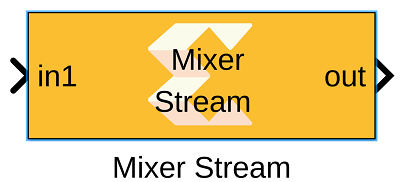# Mixer Stream - 2022.1 English

## Vitis Model Composer User Guide (UG1483)

Document ID
UG1483
Release Date
2022-05-26
Version
2022.1 English## Library

AI Engine/DSP/Stream IO

## Description

This block implements the stream-based Mixer targeted for AI Engines.

## Parameters

Main
Input/Output Data Type:
• Describes the type of individual data samples output of the DDS function. It should be `cint16`, `cint32`, or `cfloat`.
Input Frame Size (Number of Samples):
• Specifies the number of samples in the input frame. The value must be in the range `8` to `1024` and the default value is `32`.
Mixer Mode:
• This specifies the mixer operation modes. Two modes are supported by Mixer function:
Single Input Mode
This is a DDS plus Mixer for a single data input port. Each data input sample is complex multiplied with the corresponding DDS sample to create a modulated signal that is written to the output window. This is the default Mixer mode.
Dual Input Mode
This is a special configuration for symmetrical carriers and two data input ports. Each data sample of the first input is complex multiplied with the corresponding DDS sample to create a modulated signal. Each data sample of the second data input is complex multiplied with the conjugate (which is equivalent to a signal rotating in the opposite direction) of the DDS sample to create a second modulated signal. These two modulated signals are added together and written to the output window.
Number of parallel input/output (SSR):
• This parameter specifies the number of input (or output) ports and must be of the form 2^N, where N is a non-negative integer.
Phase Increment:
• Specifies the phase increment between samples. The value must be in the range `0` to `2^31` and the default value is `0`. Input value `2^31` corresponds to Pi (i.e., 180). Phase increment is calculated using the formula (Fo*(2^N)) / Fs where:
• Fo = Output frequency.
• N = 32, which represents the accumulator width, and it is fixed.
• Fs = Sampling frequency.
Initial Phase Offset:
• Specifies the initial phase offset. The default value is `0`.
• Specifies the output sampling rate of the Mixer function in Mega Samples per Second (MSPS). The value must be in the range `1` to `1000` and the default value is `200`.